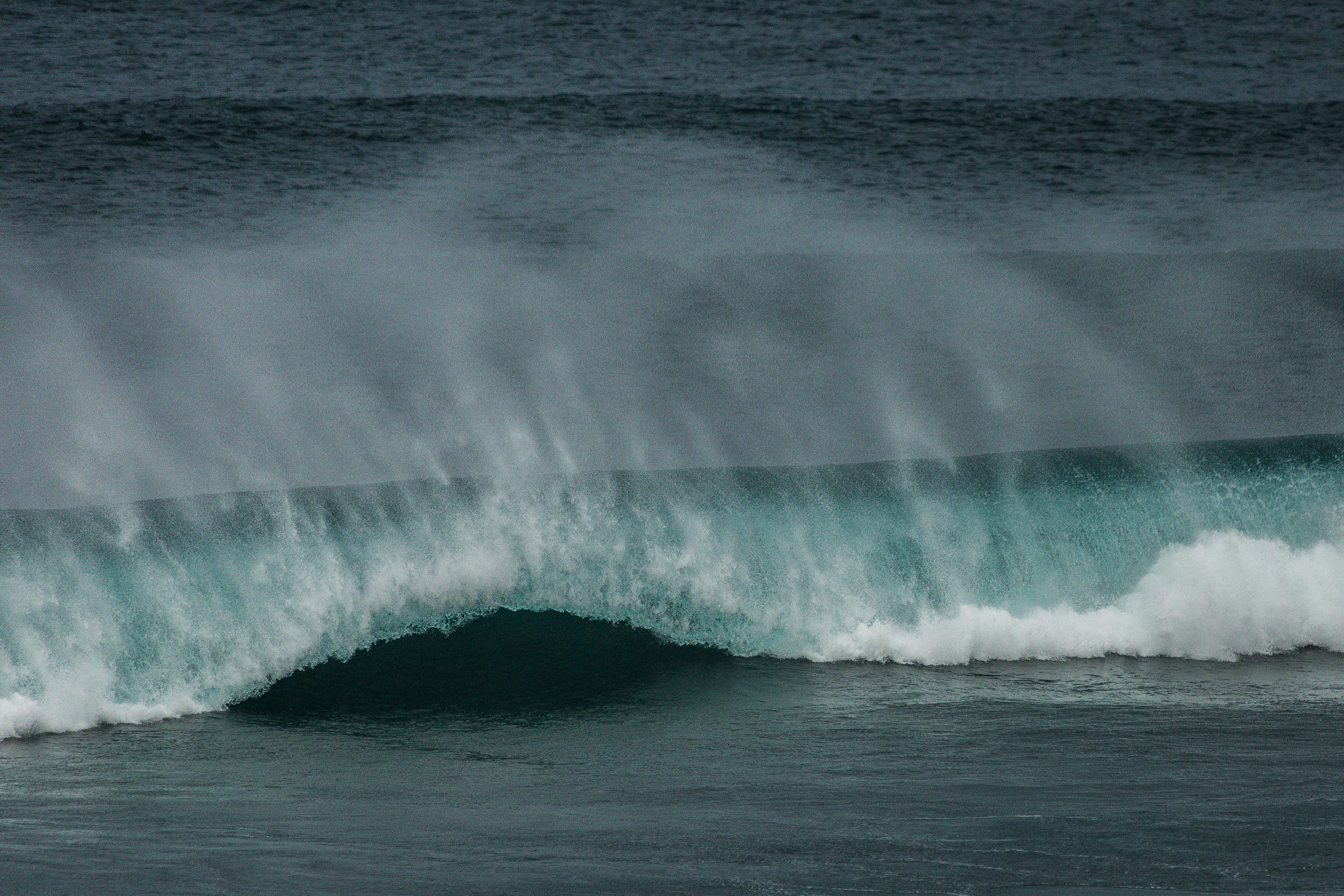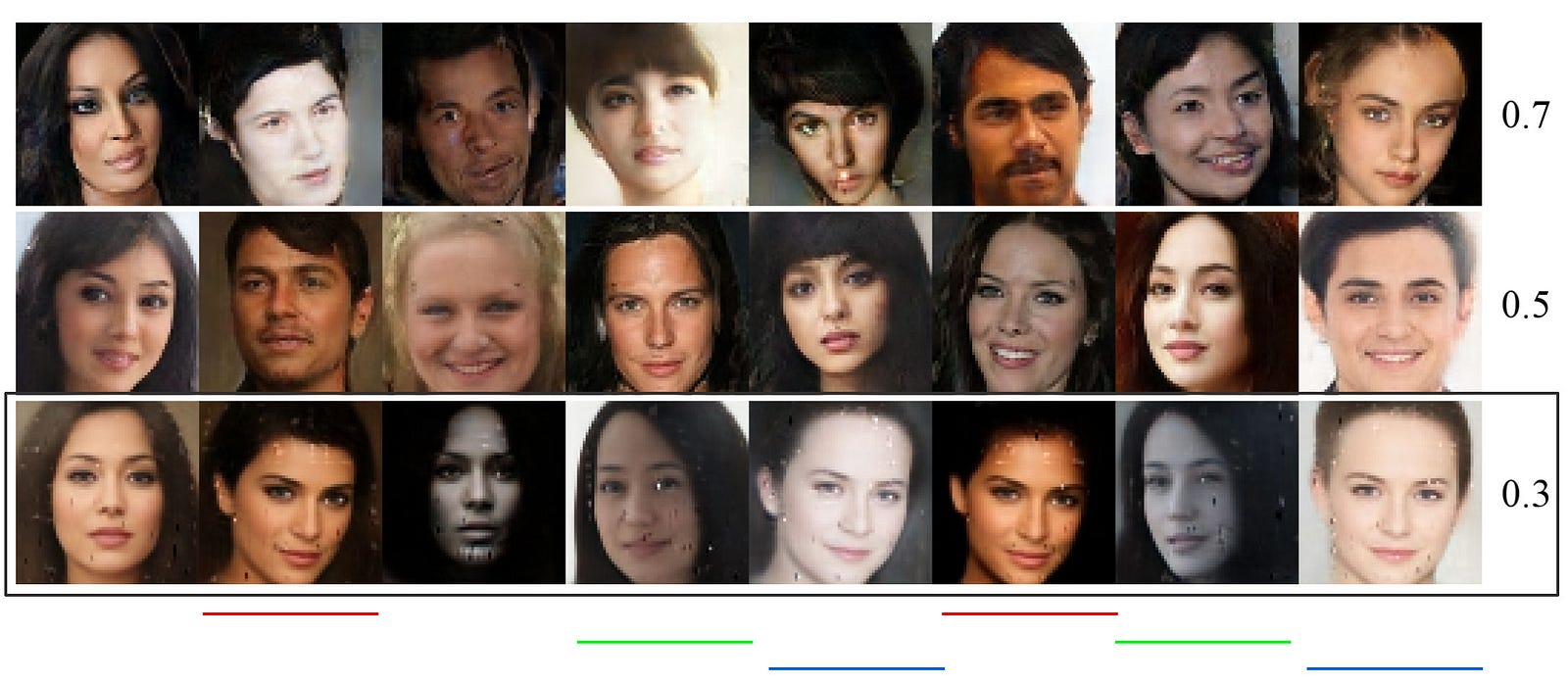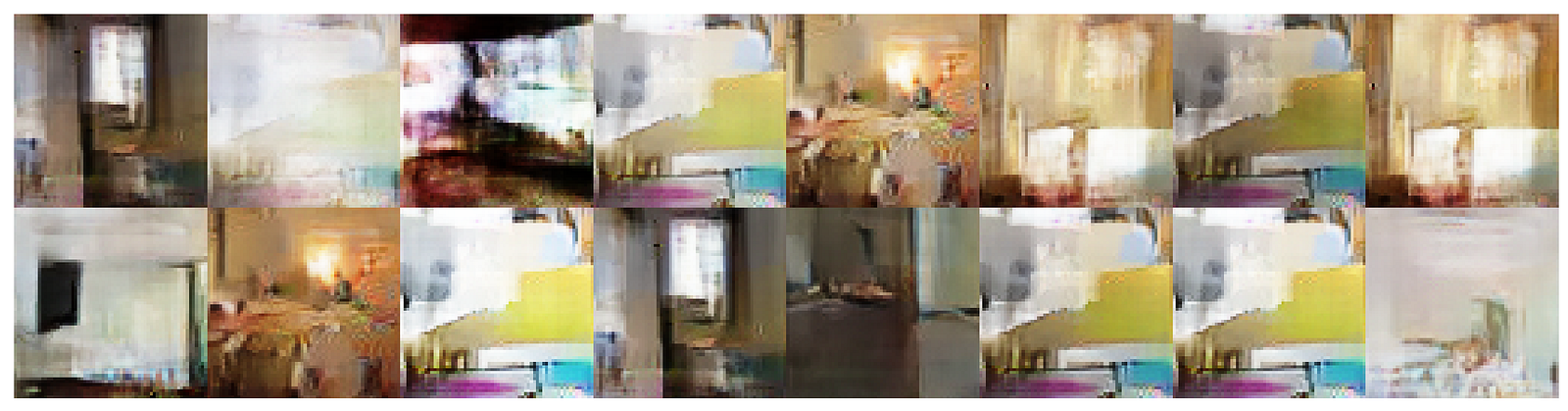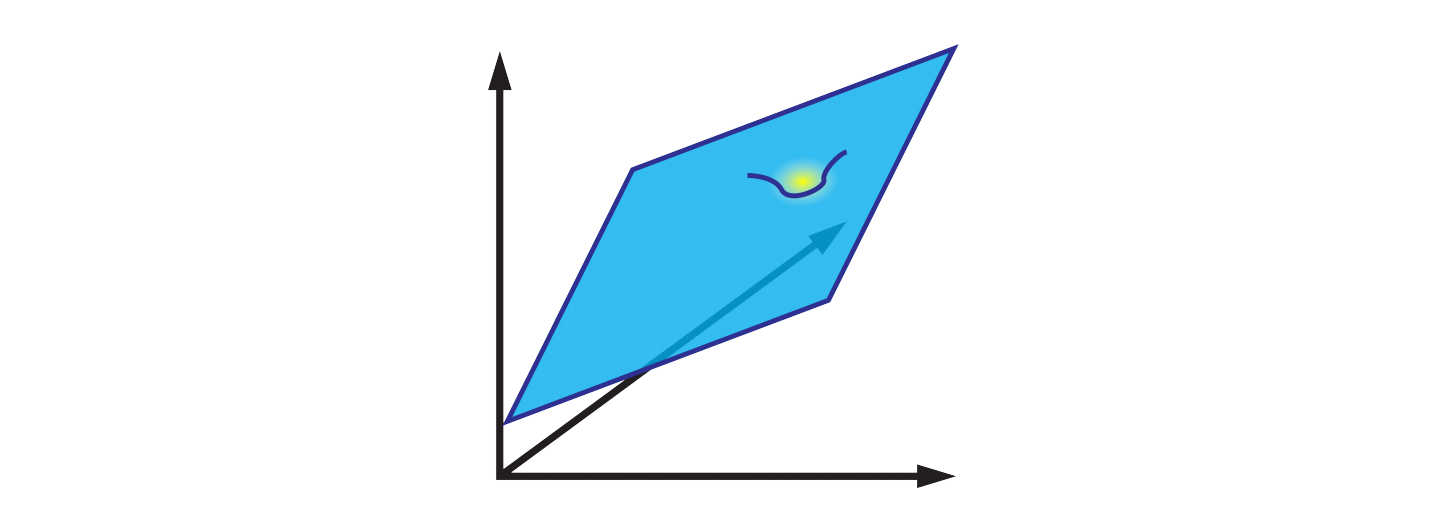# GAN — DRAGANPhoto by César Couto

Mode collapse is one major concern in training GAN. In the figure below, we use an BEGAN to generate faces. For each row, we use a different hyperparameter γ to train the model. As shown in the last row, the mode starts collapsing for γ=0.3. For example, faces underlined with the same color look similar.Modified from source.

Even though a complete collapse is not common, it remains a highly studied area:Source

As part of the GAN series, we look at the Deep Regret Analytic Generative Adversarial Networks (DRAGAN), its answer to the mode collapse and its hypothesize on the mode collapse.

### No-regret algorithm in a convex-concave game

GAN is studied as a minimax game and use the alternating gradient descent on the cost functions J to optimize the discriminator D and generator G.

In GAN, we want to find the equilibrium where the discriminator θ maximizes J and the generator ϕ minimizes it.

Let’s view it from another perspective using the regret minimization. What is the no-regret algorithm?

So what is a regret R(T). Suppose we travel from San Francisco to San Jose every day. Each day, we have a choice of taking different routes kt and use a cost function Lt to compute the cost of the route on that day. After T days, we calculate the total cost. Next, we redo the calculation but for each day, we use the same route. We compute the total cost for different routes and select the one with the lowest cost. Our regret is simply the difference.

No regret means the regret R does not grow proportional to time. i.e. the additional cost of our actions is just a fixed overhead. If T is huge, the average should approach zero.

Now we can apply no-regret learning to find the equilibrium for GAN. Let’s V* be the equilibrium value of a game (the value of J at equilibrium). For each player, we compute its average iterate defined as:

and R1 and R2 is the regret for player ϕ and θ respectively. Then it can be proven that:

If we obey the no regret condition, the regrets will approach zero and the average iterate will converge. We can solve the no regret problem using “Follow The Regularized Leader” (FTRL), i.e.

This is the online gradient descent. i.e. regret minimization leads us to the same solution for the convex-concave game that we used in GAN. Therefore, the authors use that as an alternative viewpoint in explaining the mechanics of GAN.

Consider the objective function L=xy, which one player controls x to maximize L and one player controls y to minimize L, this minimax game will not converge using the alternative gradient descent (as detailed in one of the previous GAN article).

However, a solution can be found by simply averaging the iterates in the regret minimization.

### Non-convex game

For a non-convex game, the model can converge to some local equilibrium.Source
The DRAGAN paper hypothesizes that the mode collapse is the result of the game converging to bad local equilibria.

It also hypothesizes that the sudden drop in inception score is related to the gradient’s norm. Hence, to improve image quality, DRAGAN recommends adding gradient penalty in the cost function.

### Gradient penalties

Here is the recommended gradient penalty.

where λ is about 10, k = 1 and c is about 10 for the experiments done by DRAGAN.

### More thoughts

DRAGAN suggests a new perspective in interpreting GAN. It hypothesizes that the mode collapse is the result of the game converging to bad local equilibria. To mitigate that, a gradient penalty is suggested. DRAGAN is also one of the commonly mentioned cost function in the GAN training.

To close our thoughts, we will quote one of the paper’s reviewer:

“It is OK to form the hypothesis and present an interesting research direction, but in order to make this as a main point of the paper, the author should provide more rigorous arguments or experimental studies...”

The authors have reviewed the paper accordingly but we feel the hypotheses still need more rigorous arguments as suggested by the reviewer. For more information on this point, we suggest reading the review and the response here.